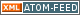www.LinuxHowtos.org howtos, tips&tricks and tutorials for linuxfrom small one page howto to huge articles all in one place

search text in:

Poll
Which filesystem do you use?

poll results

using iotop to find disk usage hogs

using iotop to find disk usage hogs

words:

887

views:

144254

userrating:

average rating: 1.8 (88 votes) (1=very good 6=terrible)

May 25th. 2007:
Words

486

Views

243488

Workaround and fixes for the current Core Dump Handling vulnerability affected kernels

Workaround and fixes for the current Core Dump Handling vulnerability affected kernels

words:

161

views:

126384

userrating:

average rating: 1.3 (28 votes) (1=very good 6=terrible)

April, 26th. 2006:

You are here: manpages

# TGAMMA

Section: Linux Programmer's Manual (3)
Updated: 2017-09-15

## NAME

tgamma, tgammaf, tgammal - true gamma function

## SYNOPSIS

#include <math.h>

double tgamma(double x);
float tgammaf(float x);
long double tgammal(long double x);

Feature Test Macro Requirements for glibc (see feature_test_macros(7)):

tgamma(), tgammaf(), tgammal():

_ISOC99_SOURCE || _POSIX_C_SOURCE >= 200112L

## DESCRIPTION

These functions calculate the Gamma function of x.

The Gamma function is defined by

Gamma(x) = integral from 0 to infinity of t^(x-1) e^-t dt

It is defined for every real number except for nonpositive integers. For nonnegative integral m one has

Gamma(m+1) = m!

and, more generally, for all x:

Gamma(x+1) = x * Gamma(x)

Furthermore, the following is valid for all values of x outside the poles:

Gamma(x) * Gamma(1 - x) = PI / sin(PI * x)

## RETURN VALUE

On success, these functions return Gamma(x).

If x is a NaN, a NaN is returned.

If x is positive infinity, positive infinity is returned.

If x is a negative integer, or is negative infinity, a domain error occurs, and a NaN is returned.

If the result overflows, a range error occurs, and the functions return HUGE_VAL, HUGE_VALF, or HUGE_VALL, respectively, with the correct mathematical sign.

If the result underflows, a range error occurs, and the functions return 0, with the correct mathematical sign.

If x is -0 or +0, a pole error occurs, and the functions return HUGE_VAL, HUGE_VALF, or HUGE_VALL, respectively, with the same sign as the 0.

## ERRORS

See math_error(7) for information on how to determine whether an error has occurred when calling these functions.

The following errors can occur:

Domain error: x is a negative integer, or negative infinity
errno is set to EDOM. An invalid floating-point exception (FE_INVALID) is raised (but see BUGS).
Pole error: x is +0 or -0
errno is set to ERANGE. A divide-by-zero floating-point exception (FE_DIVBYZERO) is raised.
Range error: result overflow
errno is set to ERANGE. An overflow floating-point exception (FE_OVERFLOW) is raised.

glibc also gives the following error which is not specified in C99 or POSIX.1-2001.

Range error: result underflow
An underflow floating-point exception (FE_UNDERFLOW) is raised, and errno is set to ERANGE.

## VERSIONS

These functions first appeared in glibc in version 2.1.

## ATTRIBUTES

For an explanation of the terms used in this section, see attributes(7).
 Interface Attribute Value tgamma(), tgammaf(), tgammal() Thread safety MT-Safe

## CONFORMING TO

C99, POSIX.1-2001, POSIX.1-2008.

## NOTES

This function had to be called "true gamma function" since there is already a function gamma(3) that returns something else (see gamma(3) for details).

## BUGS

Before version 2.18, the glibc implementation of these functions did not set errno to EDOM when x is negative infinity.

Before glibc 2.19, the glibc implementation of these functions did not set errno to ERANGE on an underflow range error. x

In glibc versions 2.3.3 and earlier, an argument of +0 or -0 incorrectly produced a domain error (errno set to EDOM and an FE_INVALID exception raised), rather than a pole error.

gamma(3), lgamma(3)

## Index

NAME
SYNOPSIS
DESCRIPTION
RETURN VALUE
ERRORS
VERSIONS
ATTRIBUTES
CONFORMING TO
NOTES
BUGS
COLOPHON||- Powered by- Running on-
Copyright 2004-2020 Sascha Nitsch Unternehmensberatung GmbH::- Level Triple-A Conformance to Web Content Accessibility Guidelines 1.0 -
- Copyright and legal notices -